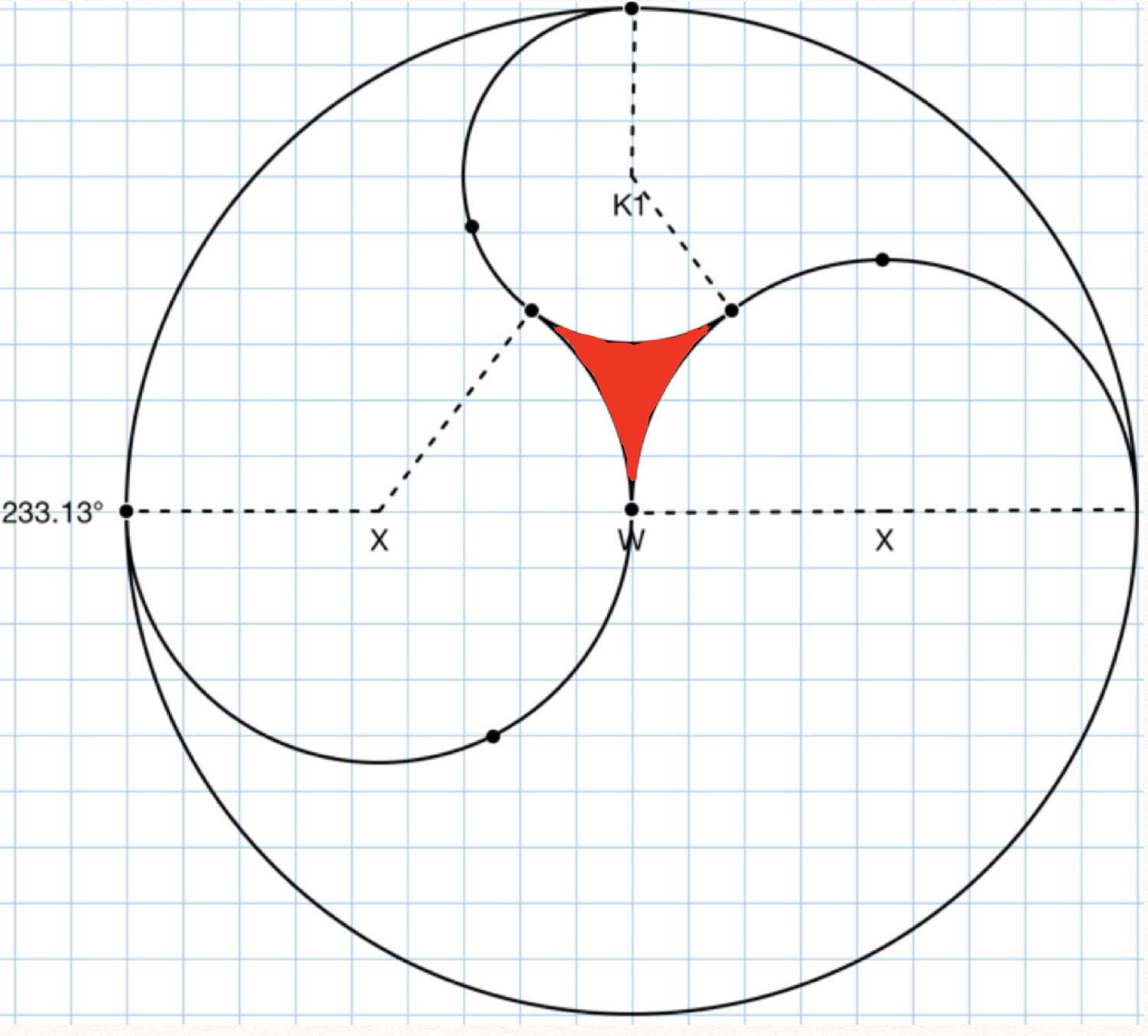# Extreme Yin-Yang 2

Geometry Level 5The figure above is created by three tear drop shaped figures. If the large circle has a radius of 9 units, find the area of the red triangular sector in the middle of the circle.

Assume:

• The tear drops have circular heads.

• You may use a calculator for operations such as +, -, /,*.

• The two arcs with centers labeled x have the same radius and are tangent along the diameter of the largest circle.

• Cos(53.13)=0.6

Challenge: see if you can solve this problem without the degree measure on the left of the figure.

×